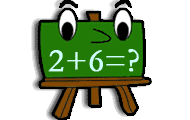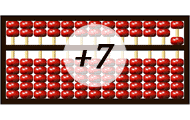Filtering on knowledge field: Addition

Math games that will teach you about mathematics, numbers and counting. Practice the basic number perception with an abacus, or practice the four rules of arithmetic. You will learn math in a joyful way by playing these games. Here we have collected all the games on the subject Math. The games suit preschool, elementary school and all the way up to high school. The games may be sorted by popularity, target group, release date or name.
0%## Number bonds

• Numbers
• Mental calculation
• Number bonds
Try to find the number that is a number bond to another number and adds up to the given sum. Practice part-part-whole relationships to the numbers 0-20 and facilitates the important mental calculation
0%• Numbers
• Mental calculation
Practice addition in math game with several levels of increasing difficulty. Add two numbers and find the correct sum.
0%